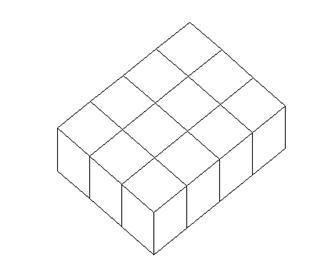### Sample Problem

Solid A and solid B are made of 1- inch cubes.

The volume of solid A is inch3. The volume of solid B is inch3.AB

#### Solution

Va = 4 × 3 × 1 = 12

Vb = 3 × 3 × 3 = 27